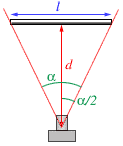# Determining the viewing angle of a camera

Because a normal camera makes a central projection on a plane, only a limited part of a scene can be photographed. The handiest parameter to describe the viewable area in a universal manner is the viewing angle, also called field-of-view.
The horizontal viewing angle, or field-of-view, of an optical device is the angle between the left edge of the viewable region, the eyepoint and the right edge. The vertical viewing angle is the angle between the top edge, the eyepoint and the and bottom edge.

With a simple measurement and some simple goniometrics, we can calculate the viewing angle of any camera which has a viewer based on mirrors so that the preview you get, is exactly what will be photographed. The only thing you need is a measuring tape and a calculator which can calculate the inverse tangent (arctan, atan) of a number.You need to start from a reference object with a width l that can be measured, or you can simply mark a chosen distance on a wall in front of you. Next, look through your camera towards the object, and make sure you are looking perpendicularly towards it, like in the image at the right. Next, move backwards or forwards until the object fits exactly within the viewable area of your camera. Now you must measure the perpendicular distance between the camera and the object, called d in the image. This may not be that simple, so you could ask a second person to do it, or you could do the measurement while lying on the ground so you can simply put the camera on the ground without making a large error.
To be exact, you should measure the distance between the object and the focal point of the camera, but because it is hard to determine this point exactly, you can ignore the error introduced by this simplification. This simplification is only valid if you work with rather large distances, this means: at least a few meters for d.

So now you have the two distances l and d from the figure. With some simple goniometry, one can obtain:

tan(α/2) = (l/2)/d,

hence

α = 2*atan(l/(2d))

So with this formula you can calculate the horizontal field-of-view of your camera. Of course measuring the vertical f.o.v. goes exactly the same way except that you then need to view the object in its vertical position.

For the panorama, we are interested in the smallest field-of-view, which is the horizontal field-of-view in “portrait” mode.